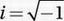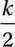# SAT Math Multiple Choice Question 642: Answer and Explanation

### Test Information

Question: 642

12. If i2k = 1, and, which of the following must be true about k?

• A. k is a multiple of 4.
• B. k is a positive integer.
• C. When 2k is divided by 4, the remainder is 1.
• D.is an integer.